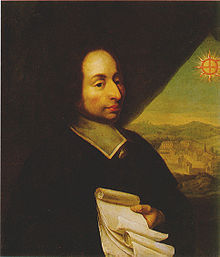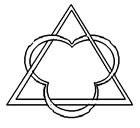Posted on

# Pascal’s TrianglePascal, Blaise (1623-1662)

Pascal’s Triangle was originally developed by the ancient Chinese, but Blaise Pascal was the first person to discover special patterns contained inside the triangle. They teach his ideas in various schools online in math courses. You probably also heard of this guy from your high school math teacher.

Triangular numbers appear in Pascal’s Triangle. In fact, the 3rd diagonal of Pascal’s Triangle gives all triangular numbers as shown below:

1
1 1
1 2 1
1 3 3 1
1 4 6 4 1
1 5 10 10 5 1
1 6 15 20 15 6 1
1 7 21 35 35 21 7 1
1 8 28 56 70 56 28 8 1
1 9 36 84 126 126 84 36 9 1

At the tip of Pascal’s Triangle is the number 1, which makes up the zeroth row. The first row (1 & 1) contains two 1’s, both formed by adding the two numbers above them to the left and the right, in this case 1 and 0 (all numbers outside the Triangle are 0’s). Do the same to create the 2nd row: 0+1=1; 1+1=2; 1+0=1. And the third: 0+1=1; 1+2=3; 2+1=3; 1+0=1. In this way, the rows of the triangle go on infinitly. A number in the triangle can also be found by nCr (n Choose r) where n is the number of the row and r is the element in that row. For example, in row 3, 1 is the zeroth element, 3 is element number 1, the next three is the 2nd element, and the last 1 is the 3rd element. The formula for nCr is:

n!
——–
r!(n-r)!

! means factorial, or the preceeding number multiplied by all the positive integers that are smaller than the number. 5! = 5 × 4 × 3 × 2 × 1 = 120.

The sum of the numbers in any row is equal to 2 to the nth power or 2n, when n is the number of the row. For example:

 20 = 121 = 1+1 = 222 = 1+2+1 = 423 = 1+3+3+1 = 824 = 1+4+6+4+1 = 16

###Fibonnacci’s Sequence can also be located in Pascal’s Triangle. The sum of the numbers in the consecutive rows shown in the diagram are the first numbers of the Fibonnacci Sequence. The Sequence can also be formed in a more direct way, very similar to the method used to form the Triangle, by adding two consecutive numbers in the sequence to produce the next number. The creates the sequence: 1,1,2,3,5,8,13,21,34, 55,89,144,233, etc . . . . The Fibonnacci Sequence can be found in the Golden Rectangle, the lengths of the segments of a pentagram, and in nature, and it decribes a curve which can be found in string instruments, such as the curve of a grand piano. The formula for the nth number in the

Fibonnacci Sequence is 1,1,2,3,5,8,13,21,… where each new element is one plus the preceding elament.

### Connection to Serpinski’s triangle

 When all the odd numbers (numbers not divisible by 2) in Pascal’s Triangle are filled in (black) and the rest (the evens) are left blank (white), the recursive Sierpinski Triangle fractal is revealed (see figure at near right), showing yet another pattern in Pascal’s Triangle. Other interesting patterns are formed if the elements not divisible by other numbers are filled, especially those indivisible by prime numbers.